Documentation

## Effects of Time-Varying Source Blocks on Frequency Response Estimation

### Setting Time-Varying Sources to Constant for Estimation Using Linear Analysis Tool

This example illustrates the effects of time-varying sources on estimation. The example also shows how to set time-varying sources to be constant during estimation to improve estimation results.

1. Open the Simulink® model.

```sys = 'scdspeed_ctrlloop'; open_system(sys)```
2. Linearize the model.

1. Set the `Engine Model` block to normal mode for accurate linearization.

`set_param('scdspeed_ctrlloop/Engine Model','SimulationMode','Normal')`

2. Open the Linear Analysis Tool for the model.

In the Simulink model window, in the Apps gallery, click Model Linearizer.

3. ClickBode to linearize the model and generate a Bode plot of the result.

The linearized model, `linsys1`, appears in the Linear Analysis Workspace.

3. Create an input sinestream signal for the estimation.

1. Open the Create sinestream input dialog box.

In the Estimation tab, in the Input Signal drop-down list, select Sinestream.

2. Open the Add frequencies dialog box.

Click.

3. Specify the input sinestream frequency range and number of frequency points.

Enter `10` in the From box.

Enter `100` in the To box.

Enter `10` in the box for the number of frequency points.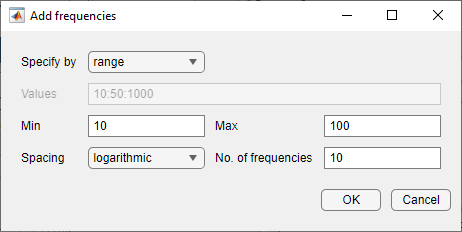Click .

The added points are visible in the Frequency content viewer of the Create sinestream input dialog box.

4. In the Frequency content viewer of the Create sinestream input dialog box, select all the frequency points.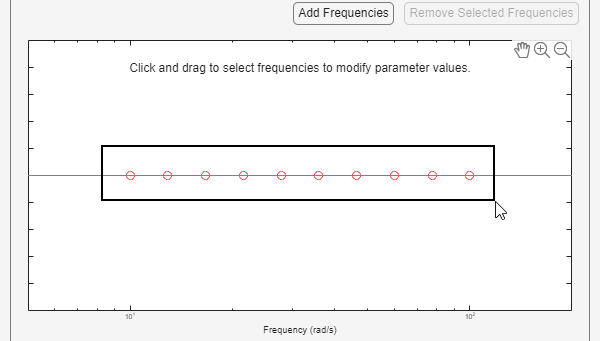5. Specify input sinestream parameters.

Change the Number of periods and Settling periods to ensure that the model reaches steady-state for each frequency point in the input sinestream.

Enter `30` in the Number of periods box.

Enter `25` in the Settling periods box.

6. Create the input sinestream.

Click . The new input signal, `in_sine1`, appears in the Linear Analysis Workspace.

4. Set the Diagnostic Viewer to open when estimation is performed.

Select the Launch Diagnostic Viewer check box.

5. Estimate the frequency response for the model.

ClickBode Plot 1 to estimate the frequency response. The Diagnostic Viewer appears in the plot plane and the estimated system `estsys1`, appears in the Linear Analysis Workspace.

6. Compare the estimated model and the linearized model.

1. Click on the Diagnostic Viewer - estsys1 tab in the plot area of the Linear Analysis Tool.

2. Click and drag `linsys1` onto the Diagnostic Viewer to add `linsys1` to the Bode Diagram.

3. Click the Diagnostic Viewer tab.4. Configure the Diagnostic Viewer to show only the frequency point where the estimation and linearization results do not match.

In the Frequency Selector section, enter `9` in the From box and `11` in the To box to set the frequency range that is analyzed in the Diagnostic Viewer.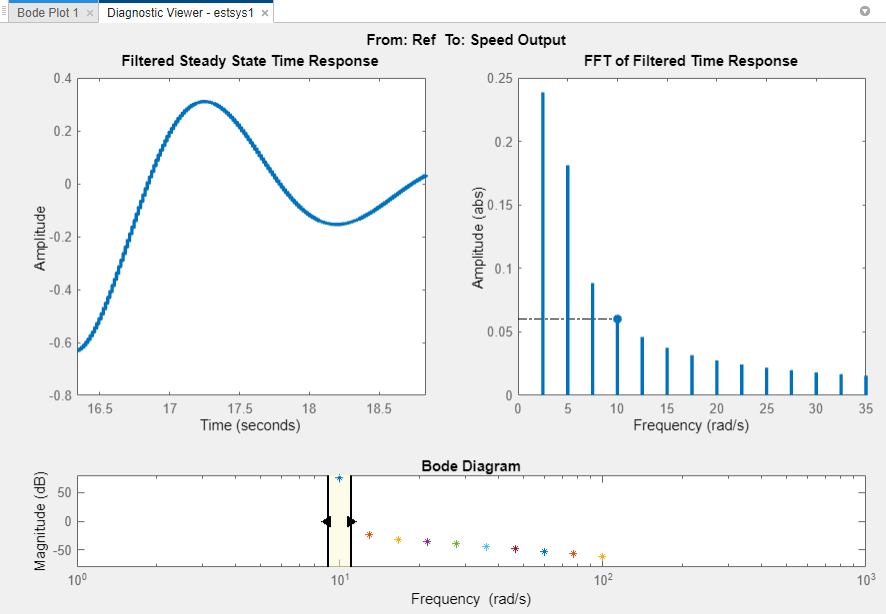The Filtered Steady State Time Response plot shows a signal that is not sinusoidal.

5. View the unfiltered time response.

Right-click the Filtered Steady State Time Response plot and clear the Show filtered steady state output only option.The step input and external disturbances drive the model away from the operating point that the linearized model uses. This prevents the response from reaching steady-state. To correct this problem, find and disable the time-varying source blocks that interfere with the estimation. Then estimate the frequency response of the model again.

7. Find and disable the time-varying sources within the model.

1. Open the Options for frequency response estimation dialog box.

On the Estimation tab, in the Options section, click More Options.2. In the Time Varying Sources tab, click .

This action populates the time varying sources list with the block paths of the time varying sources in the model. These sources will be held constant during estimation.8. Estimate the frequency response for the model.

ClickBode Plot 1 to estimate the frequency response. The estimated system `estsys2`, appears in the Linear Analysis Workspace.

9. Compare the newly estimated model and the linearized model.

Click on the Diagnostic Viewer - estsys2 tab in the plot area of the Linear Analysis Tool.

Click and drag `linsys1` onto the Diagnostic Viewer.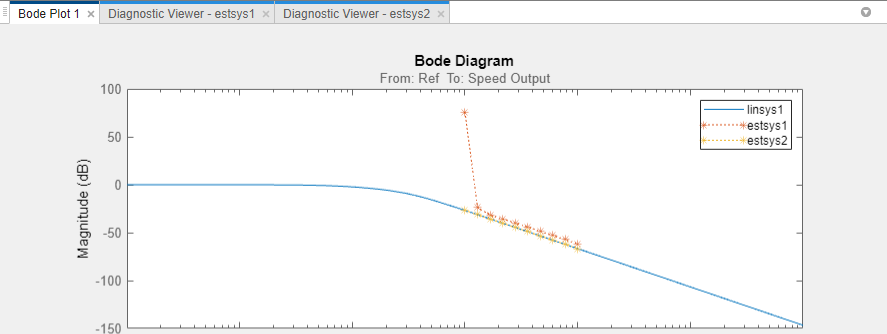The frequency response obtained by holding the time-varying sources constant matches the exact linearization results.

### Setting Time-Varying Sources to Constant for Estimation (MATLAB Code)

Compare the linear model obtained using exact linearization techniques with the estimated frequency response:

```% Open the model mdl = 'scdspeed_ctrlloop'; open_system(mdl) io = getlinio(mdl); % Set the model reference to normal mode for accurate linearization set_param('scdspeed_ctrlloop/Engine Model','SimulationMode','Normal') % Linearize the model sys = linearize(mdl,io); % Estimate the frequency response between 10 and 100 rad/s in = frest.Sinestream('Frequency',logspace(1,2,10),'NumPeriods',30,'SettlingPeriods',25); [sysest,simout] = frestimate(mdl,io,in); % Compare the results frest.simView(simout,in,sysest,sys)```

The linearization results do not match the estimated frequency response for the first two frequencies. To view the unfiltered time response, right-click the time response plot, and clear Show filtered steady state output only.The step input and external disturbances drive the model away from the operating point, preventing the response from reaching steady-state. To correct this problem, find and disable these time-varying source blocks that interfere with the estimation.

Identify the time-varying source blocks using `frest.findSources`.

`srcblks = frest.findSources(mdl,io);`

Create a `frestimate` options set to disable the blocks.

```opts = frestimateOptions; opts.BlocksToHoldConstant = srcblks;```

Repeat the frequency response estimation using the optional input argument `opts`.

```[sysest2,simout2] = frestimate(mdl,io,in,opts); frest.simView(simout2,in,sysest2,sys)```

Now the resulting frequency response matches the exact linearization results. To view the unfiltered time response, right-click the time response plot, and clear Show filtered steady state output only.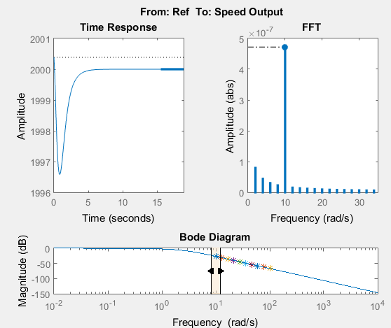##### SupportGet trial now